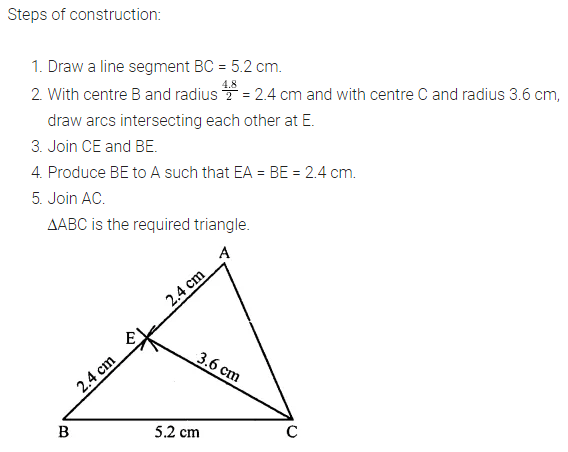# ML Aggarwal Class 7 Solutions for ICSE Maths Chapter 13 Practical Geometry Objective Type Questions

## ML Aggarwal Class 7 Solutions for ICSE Maths Chapter 13 Practical Geometry Objective Type Questions

Higher Order Thinking Skills (HOTS)

Question 1.
Construct a triangle ABC such that BC = 5.2 cm, AB = 4.8 cm and median CM = 3.6 cm.
Solution:Question 2.
Construct an isosceles right-angled triangle ABC such that its hypotenuse BC = 6 cm.
Solution: